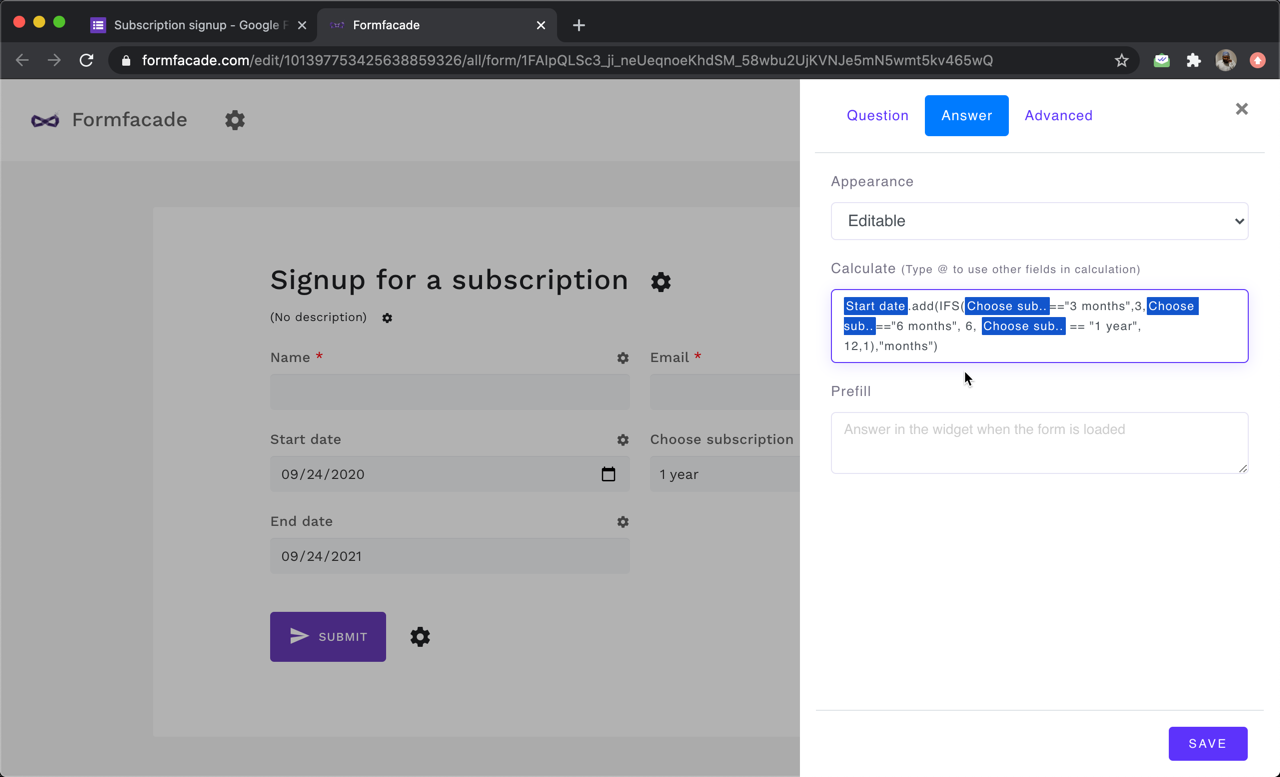# How to calculate the end date based on the start date and term duration selected by the user?

### You can use the ADD function to dynamically calculate the end date based on start date and duration selected by the user.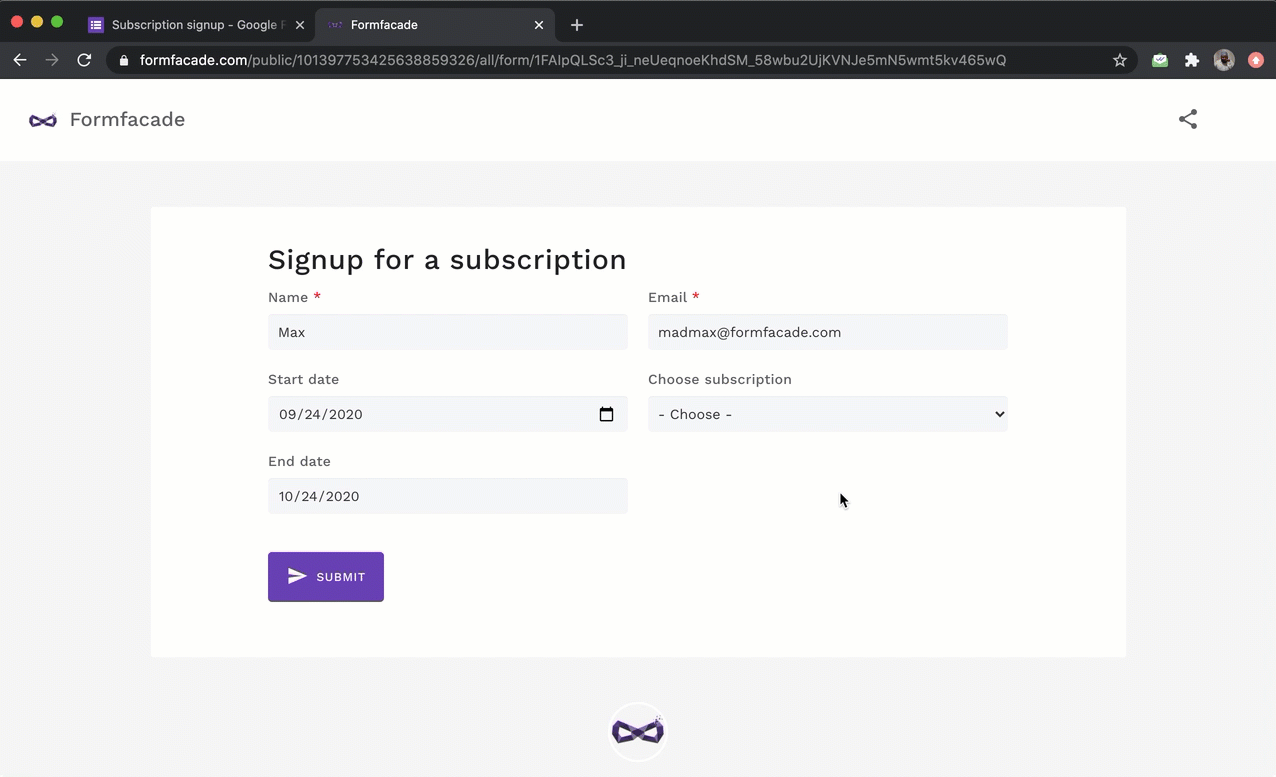Let's take the example of a subscription form. The users select the start date and their preferred subscription term, and the form should automatically display the end date based on this selection. We will use the ADD function to dynamically add the months from the subscription question to the start date entered by the user and calculate the end date.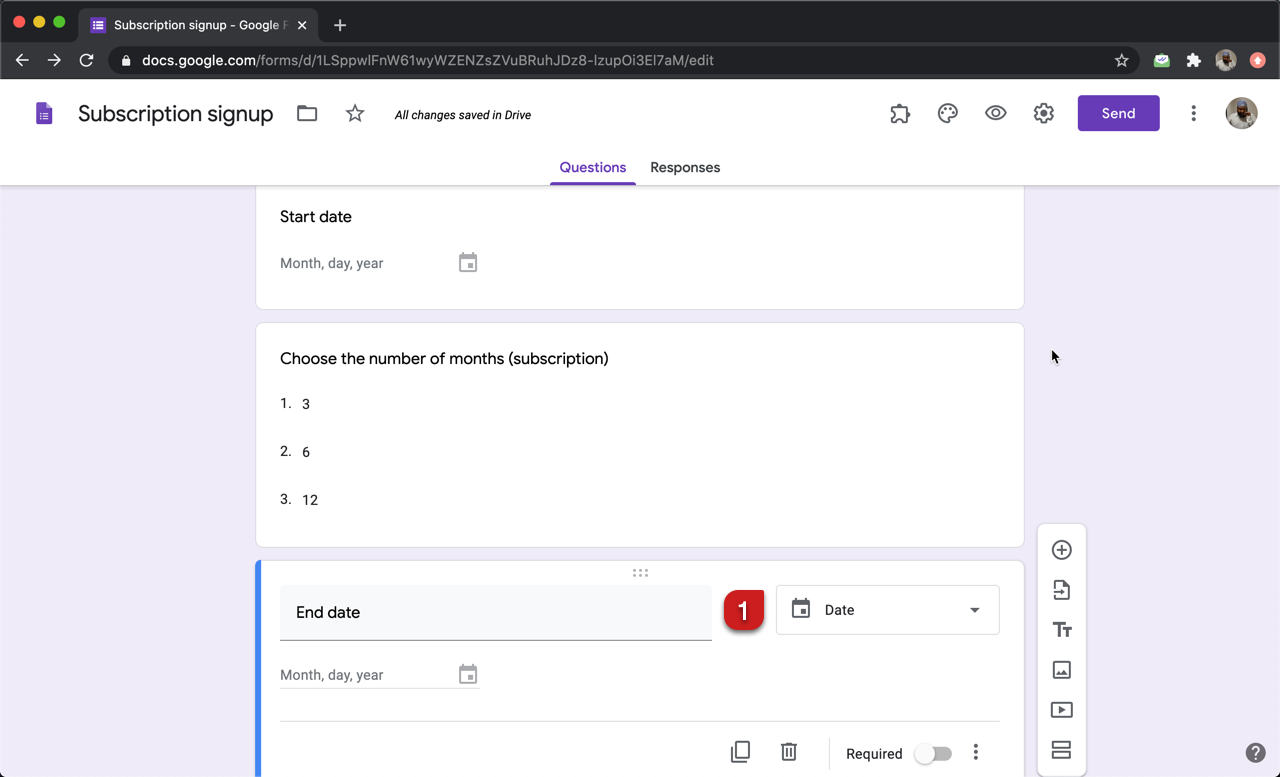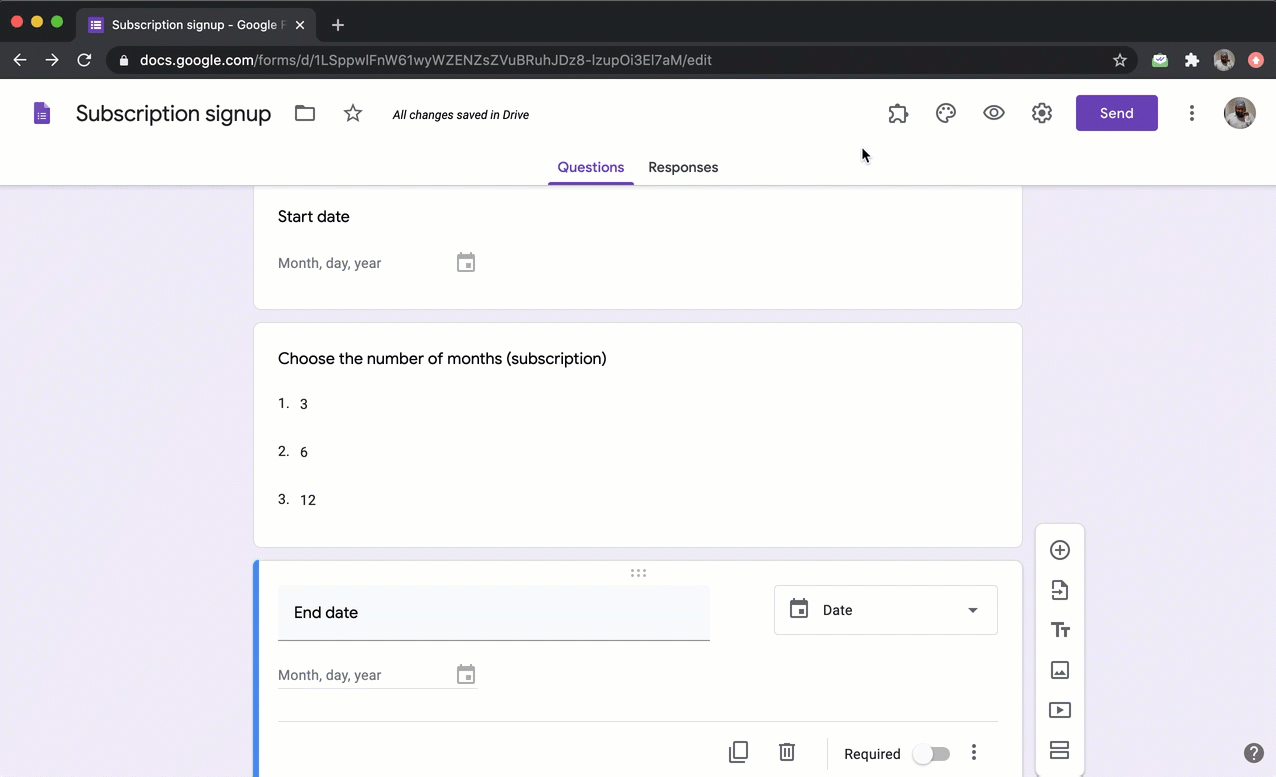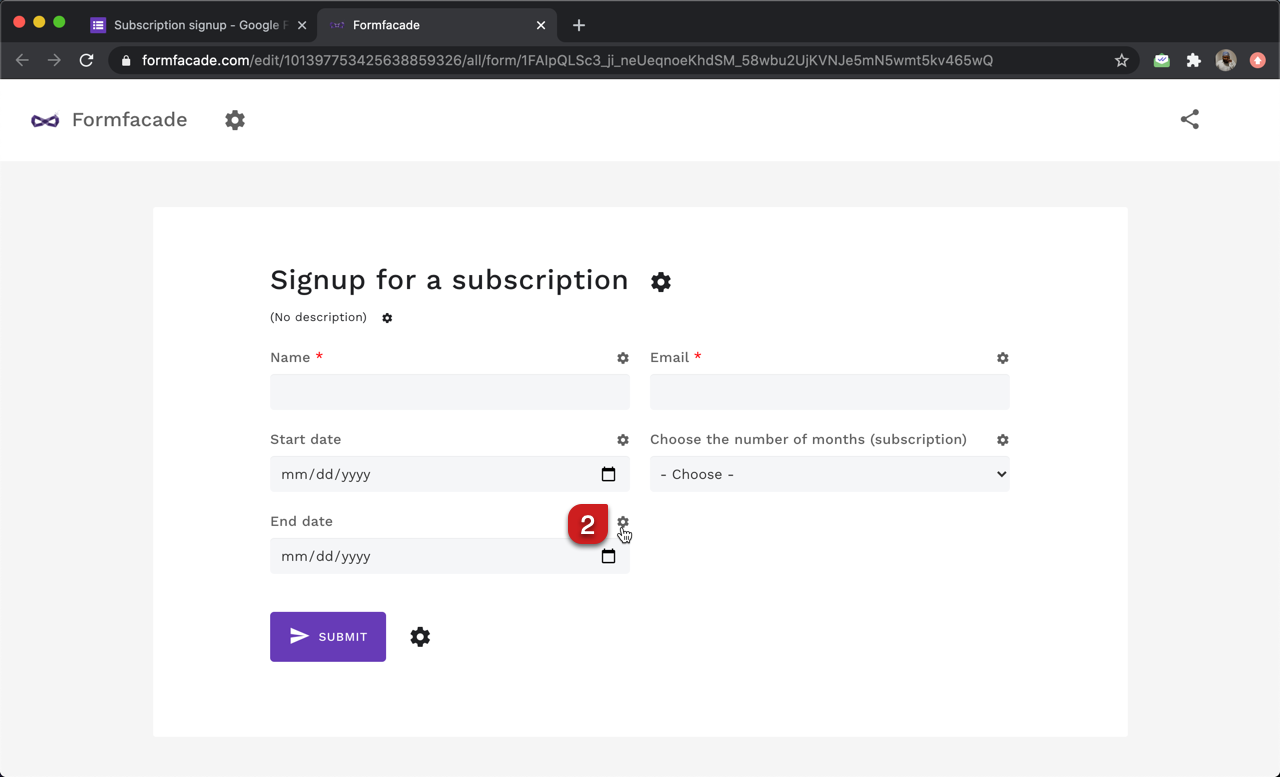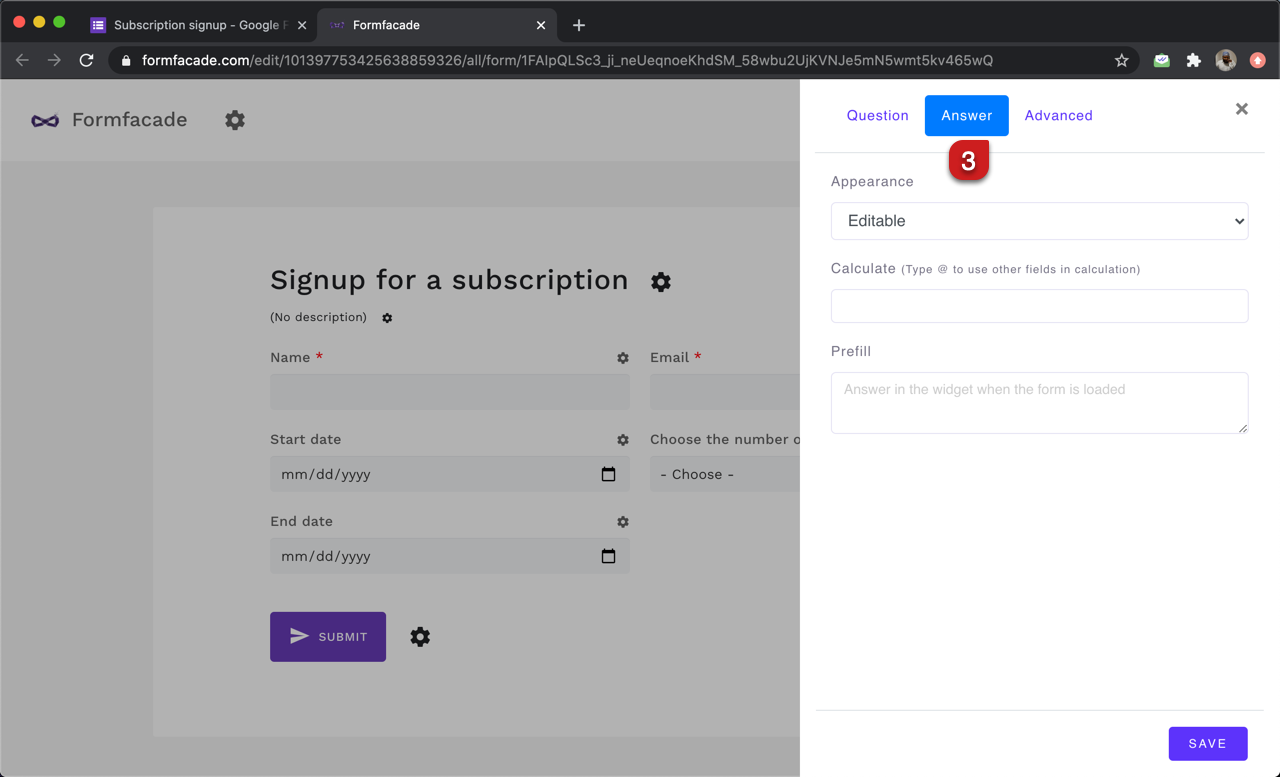#### Number answer choices in the subscription question

The subscription is a dropdown question with three number answer choices: 3, 6 and 12. We can use this directly to add the months to the start date and calculate the end date.

You can add months to a date by specifying "months" as the term in the ADD function.

Syntax:

Note: To enter start_date and number_of_months, type @ in the calculate option and select the start date and subscription question from the list.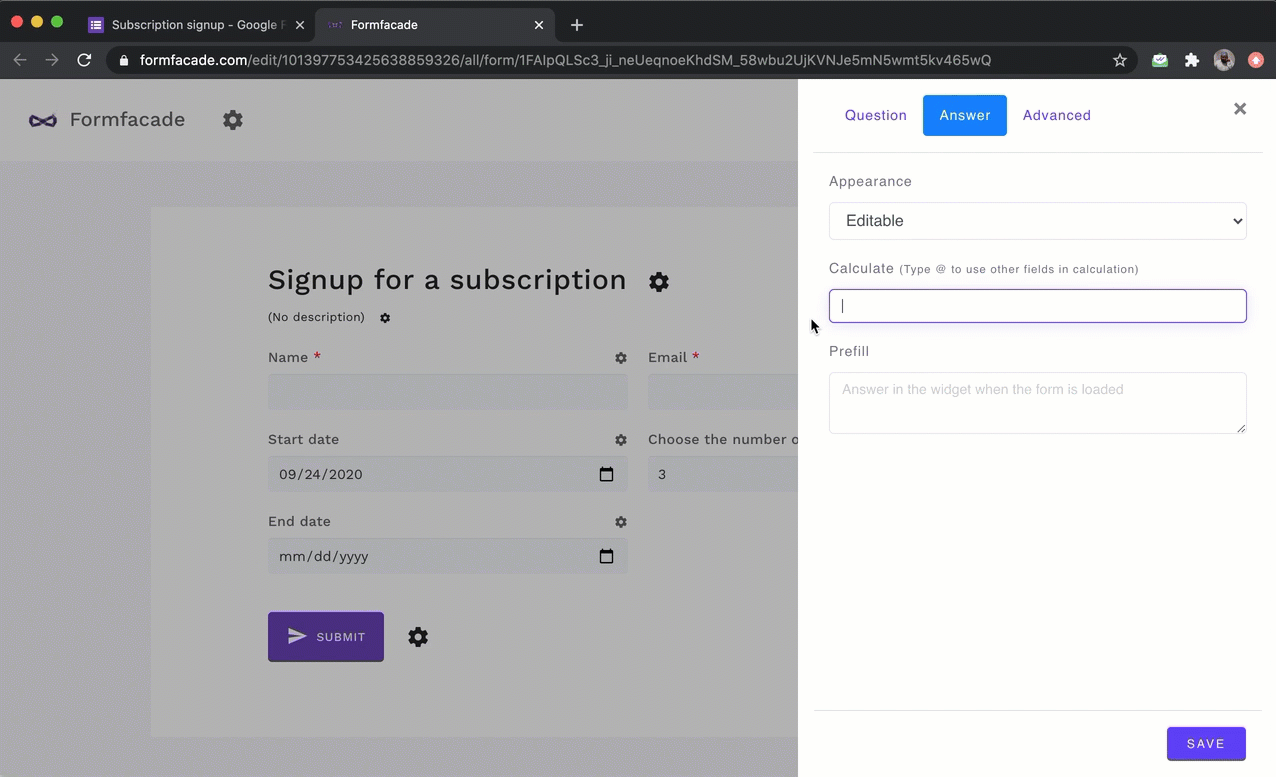#### Text answer choices in the subscription question

If you want to use text answer choices such as 3 months, 6 months and 1 year for the subscription dropdown question, you will have to use the IFS function to assign the numerical values for each of the the text answer choices. For example:

start_date.add(IFS(subscription == "3 months", 3, subscription == "6 months", 6, subscription == "1 year", 12, 1),"months")

Note: To enter start_date and subscription, type @ in the calculate option and select the start date and subscription question from the list.